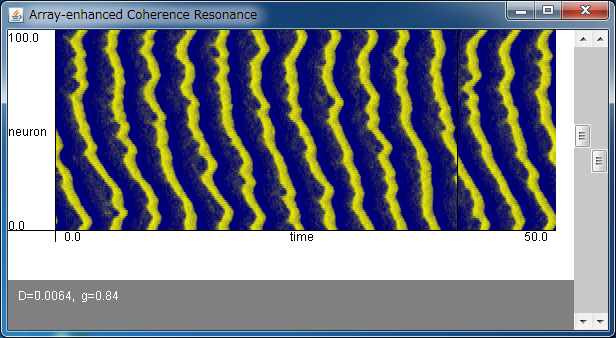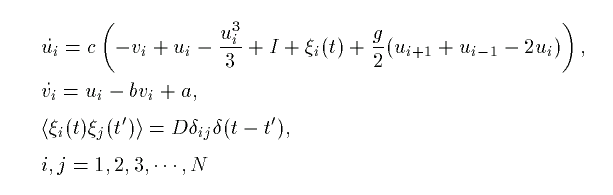# Array-Enhanced Coherence ResonanceIf the above application does not start, please install OpenJDK from adoptium.net.

Let us consider the coupled FitzHugh-Nagumo equations written aswhere a, b, c, and I are parameters and fixed as a=0.7, b=0.8, c=10.0, and I=0.3.
This is a model of a chain of neurons electrically coupled with gap junctions.

With the above applet, you can regulate the noise intensity D and the coupling strength g and observe the dynamics of the system.
The horizontal and vertical axes denote the time and the index of neurons, respectively. The number of neurons is fixed as N=100.
The plane is colored according to the state ui of each neuron. The blue color denotes small ui, and red color means that the neuron is firing.

As you can see by regulating D and g with the scroll bars, the firings of each neuron become regular for appropriately chosen D and g.
This means that the periodic solution is emerged with noise. Particularly, the couplings of elements help the propagation of pulses and, as a result, the firings of each neuron becomes more regular.
Such a phenomenon is called array-enhanced coherence resonance.

By observing the ratio of the mean to the standard error of the period of the firings,
the regularity, or, the coherence of the firings is maximized at about g=0.4 and D=0.004.

In this model, the neuron is coupled only with the nearest ones. When the coupling is all-to-all, the theoretical analysis is possible in the limit of large N, and it is concluded that array-enhanced coherence resonance is caused by bifurcations with g and D.